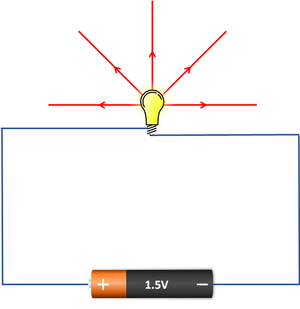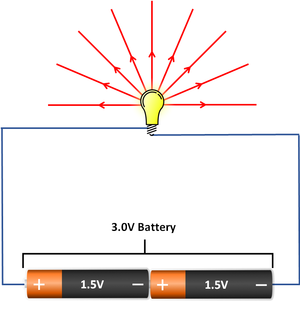# Potential Difference

## Key Stage 2

### Meaning

Voltage is how much push electricity has.

The bigger the voltage the more push the electricity has to go around the circuit.
Adding another cell to the circuit in series will increase the voltage.
With a bigger voltage a lamp will be brighter and a buzzer will be louder.A series circuit with one cell and one bulb. A bulb will be brighter if the voltage is higher.

## Key Stage 3

### Meaning

Potential Difference is how much energy is transferred by a current.

Potential Difference is measured using a Voltmeter.
The units of potential difference are Volts (V).
Potential Difference is sometimes described as the 'push' that moves a current around a circuit.

## Key Stage 4

### Meaning

Potential Difference is the amount of energy transferred per unit charge between two points in a circuit.

Potential Difference is measured using a Voltmeter.
The units of potential difference are Volts (V).
Potential difference is the difference in potential between two points in a circuit.
Potential difference can be measured between two points in a circuit and is measured across a component.
If two points in a circuit are at the same potential there is no potential difference between them so no energy is transferred between those two points.

### Equation

NB: You should remember this equation with energy transferred as the subject of the formula.

Charge = (Energy Transferred)/(Potential Difference)

$$V=\frac{E}{Q}$$

Where

$$V$$ = The potential difference between two points.

$$Q$$ = The amount of charge that move between two points.

$$E$$ = The Energy Transferred by the charge.

### Example Calculations

#### Finding Potential Difference from Charge and Energy Transferred

 A charge of 84C transfers an energy of 20kJ. Calculate the potential difference correct to two significant figures. 170J of energy is transferred by a charge of 92mC. Calculate the potential difference correct to two significant figures. 1. State the known quantities in correct units. Q = 84C E = 20kJ = 20x103J 1. State the known quantities in correct units. Q = 92mC = 92x10-3C E = 170J 2. Substitute the numbers into the equation and solve. $$V=\frac{E}{Q}$$ $$V=\frac{20 \times 10^3}{84}$$ $$V=238.0952V$$ $$V\approx 240V$$ 2. Substitute the numbers into the equation and solve. $$V=\frac{E}{Q}$$ $$V=\frac{170}{92 \times 10^{-3}}$$ $$V=1847.826V$$ $$V\approx 1800V$$

#### Finding Charge from Potential Difference and Energy Transferred

 The potential difference of 12V is placed across a resistor increasing its thermal energy store by 3.7J as a result. Calculate the charge that has flowed through the resistor in this time correct to two significant figures. A circuit transfers 2.8kJ of energy electrically to a motor. The potential difference across the motor is 1.5V. Calculate thecharge that has flowed through the motor in this time correct to two significant figures. 1. State the known quantities in correct units. V = 12V E = 3.7J 1. State the known quantities in correct units. V = 1.5V E = 2.8kJ = 2.8x103J 2. Substitute the numbers and evaluate. $$V=\frac{E}{Q}$$ $$12=\frac{3.7}{Q}$$ 2. Substitute the numbers and evaluate. $$V=\frac{E}{Q}$$ $$1.5=\frac{2.8 \times 10^3}{Q}$$ 3. Rearrange the equation and solve. $$Q=\frac{3.7}{12}$$ $$Q=0.3083C$$ $$Q\approx0.31C$$ 3. Rearrange the equation and solve. $$Q=\frac{2.8 \times 10^3}{1.5}$$ $$Q=1866.7C$$ $$Q\approx1900C$$

#### Finding Energy Transferred from Charge and Potential Difference

 A bolt of lightning with a potential difference 31,000kV transfers a charge of 15C. Calculate the energy transferred by this bolt of lightning correct to two significant figures. A 9V battery is able to mobilise a charge of 4.3kC during its operation. Calculate the total amount of energy stored in this battery correct to two significant figures. 1. State the known quantities in correct units. V = 31,000kV = 3.1x107V Q = 15C 1. State the known quantities in correct units. V = 9V Q = 4.3kC = 4.3x103 2. Substitute the numbers and evaluate. $$V=\frac{E}{Q}$$ $$3.1 \times 10^7=\frac{E}{15}$$ 2. Substitute the numbers and evaluate. $$V=\frac{E}{Q}$$ $$9 =\frac{E}{4.3 \times 10^3}$$ 3. Rearrange the equation and solve. $$E = 15 \times 3.1 \times 10^7$$ $$E = 4.65\times10^8 J$$ $$E\approx4.7\times10^8 J$$ 3. Rearrange the equation and solve. $$E = 4.3 \times 10^3 \times 9$$ $$E = 38700J$$ $$E \approx 39000 \times 10^4J$$